# Team:KU Leuven/Project/Oscillator/Modelling

iGem

### Secret garden

Congratulations! You've found our secret garden! Follow the instructions below and win a great prize at the World jamboree!

• A video shows that two of our team members are having great fun at our favourite company. Do you know the name of the second member that appears in the video?
• For one of our models we had to do very extensive computations. To prevent our own computers from overheating and to keep the temperature in our iGEM room at a normal level, we used a supercomputer. Which centre maintains this supercomputer? (Dutch abbreviation)
• We organised a symposium with a debate, some seminars and 2 iGEM project presentations. An iGEM team came all the way from the Netherlands to present their project. What is the name of their city?

Now put all of these in this URL:https://2013.igem.org/Team:KU_Leuven/(firstname)(abbreviation)(city), (loose the brackets and put everything in lowercase) and follow the very last instruction to get your special jamboree prize!### Design

Robust, colony-wide synchronisation

We transfered our oscillation model into a preliminary ODE system composing a rate equation for each of the six components of this circuit. We expanded upon this system by adding in the fact that the production of quorum-sensing molecules goes through an enzymatic step and by using an approximation of the OR gate that is easier to realize in the wet lab.

Then we wanted to define a parameter space for which these ODE systems produce oscillations. This was done by random probing a realistic range of the different parameters to test if sustained oscillations take place. The next test we performed was testing the synchronization and rapid synchronization of the most complete system. Each of the two tests were conducted with a multitude of oscillating parameter sets. We tested the synchronized oscillation when cell-to-cell variability is existent by assuming a colony of ten different cells of which each cell has different parameters. The rapid resynchronization was tested by using again a colony of ten different cells, however a number of the cells began with different initial conditions.

Our modeling showed that we had designed a very robust oscillator:

1. Our system produces sustained oscillations for a very broad range of parameters.
2. The oscillator can withstand a high amount of cell-to-cell variability.
3. Rapid resynchronization occurs even when 40% of the cells start out of sync.

To conduct our computations we made use of the Flemish Super Computer Centre (VSC), which is a partnership of the five Flemish university associations. To use this we first had to rewrite the tests so they could be run in parallel on the supercomputer cluster at our university.

For the transformation into a biological network, we refer you to the wetlab page.

#### 1.1 Exploring our possibilities

Firstly, it is important to clarify what we exactly mean with ‘parameter’. A biochemical system has a very high variability in ranges for transcription rates, translation rates, degradation rates, etc. On top of that these parameters are not perfectly quantified and are subject to changes in the conditions. We checked what part of the parameter space (Box 1: Explanation of parameter space) creates oscillations. There are possibilities for doing this in a purely mathematical manner. Tyson (2002) gives a good example of how to study systems that produce biochemical oscillations. Polynikis, Hogan and Bernardo (2009) described modelling approaches for gene regulatory networks more generally. This is typically done by investigating the eigenvalues of the Jacobian matrix of the system. This becomes increasingly more difficult when the number of parameters and variables increases. A high level of non-linearity complicates the study of the behaviour even further. In order to see what is possible we contacted Professor Dirk Roose, an expert in non-linear systems analysis. We explained him we want to investigate what parameter values create a synchronized oscillation. However, our parameter space consists of about 20 parameters that can each vary with more than a factor ten. On top of that we will have highly non-linear equations. Professor Roose told us this amount of variability would make a clean-cut mathematical examination of our model impossible . Since it is not possible to reduce our parameter space, without diverting from our goal of fully studying our system, we decided to use another strategy. We will study this enormous parameter space by generating random sets of parameters throughout this space. This offers a less theoretical, nonetheless effective means of assuring this model robustly produces oscillations.

#### Box1 | Explanation of parameter space

We will explain the concept parameter space with a simple example. Let’s say we want our iGEM team (our system) to develop a working oscillator. Two parameters of our system will determine whether this endeavour is successful. These parameters are the number of engineers in a team and the amount of coffee they consume per engineer. As to determine the parameter space we have to find relevant ranges for each of the parameters. We assume more than 10 engineers in the iGEM team is unlikely and a coffee consumption of more than 1.2 liter per day per engineer can also be discarded. The resulting parameter space for this two-parameter model can be easily visualised by a rectangle. This resulting parameter space can be probed and the parameter values we obtained were then used in a simulation which gave as an output whether an oscillator is produced or not. In the figure below we show the result of such a random probing of the parameter space. The blue dots represent unsuccessful endeavours and the orange dots successful ones.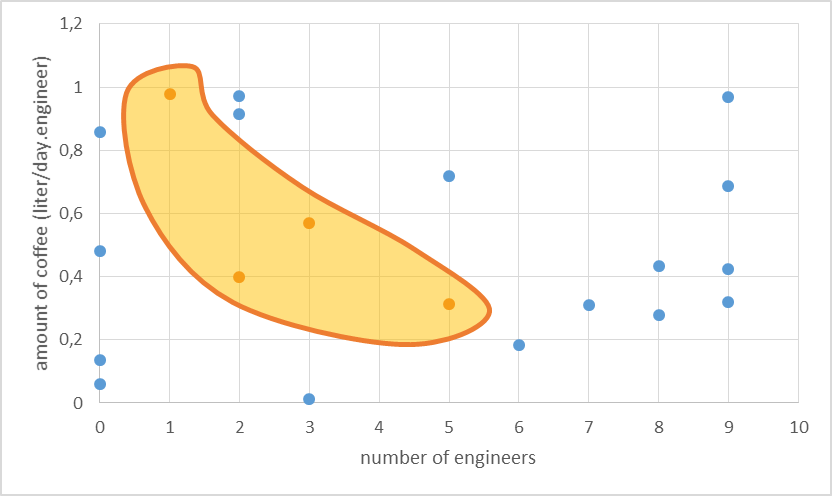The results allow us to hypothesise on what is necessary to produce an oscillator. Apparently a higher amount of engineers still makes success possible, but only when the coffee consumption is not too high. We assume here that this might be because the engineers get too much energy and start to walk around instead of being productive. We used a similar approach in the testing of our model, with some distinctions though. We deme parameter sets positive when synchronized oscillations take place. But we have 26 parameters and that makes it impossible to visualise our positive parameter space.

#### 1.2 Derivation of the ordinary differential equations (ODE’s)

##### 1.2.1 The preliminary system

The logical circuit of our oscillator is displayed in Figure 1 and for an elaborate explanation on this design we refer you to the design page. We will start with a preliminary translation of this network into a system of ODE’s, which is the most common method for modeling gene regulatory networks (Polynikis, Hogan and di Bernardo, 2009). An ODE cannot account for the fact that in reality each of the steps (like transcription and translation) take a finite amount of time. Danino et al. (2010) used delayed differential equations (DDE’s) as a solution for this problem but this complicates the mathematics enormously, especially when we want to take all our parameters into account. We will stick to ODE’s, since that should suffice for the study of our model. The extra delay should benefit the occurrence of oscillations because it helps diminishing peak overlap. The system of ODE’s is created by composing a rate equation for each of the six components of this preliminary circuit Figure 1. Those rate equations give the change of the components over time as a function of the amount of each of the components. The resulting system is displayed as Equation 1. Below we explain the meaning of the different components and afterwards we will explain each of the equations in this system. Further on we will derive the ODE systems for circuits that are slightly altered; by adding the fact that the production of quorum-sensing molecules goes through an enzymatic step and by using the implemented version of the OR gate.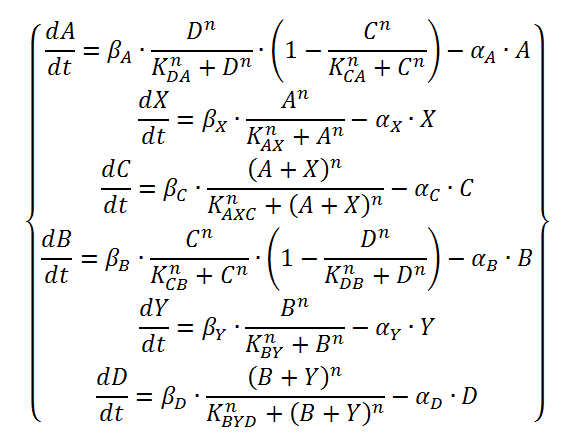Equation 1: preliminary ODE system exhibiting the behaviour of the oscillator.

The functions of the form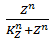are called Hill functions and are often used to represent unknown regulatory functions (Rosenfeld et al., 2005). With a high Hill coefficient, represented by n in the equations above, this function approximates a step function, as can be seen in Figure 2. The other parameter of the Hill function, indicated by K, gives the moment at which half of the maximum is reached. In the ideal case of a step function, this K value gives the threshold, above which the Hill function returns 1 and below that value, the Hill function returns 0.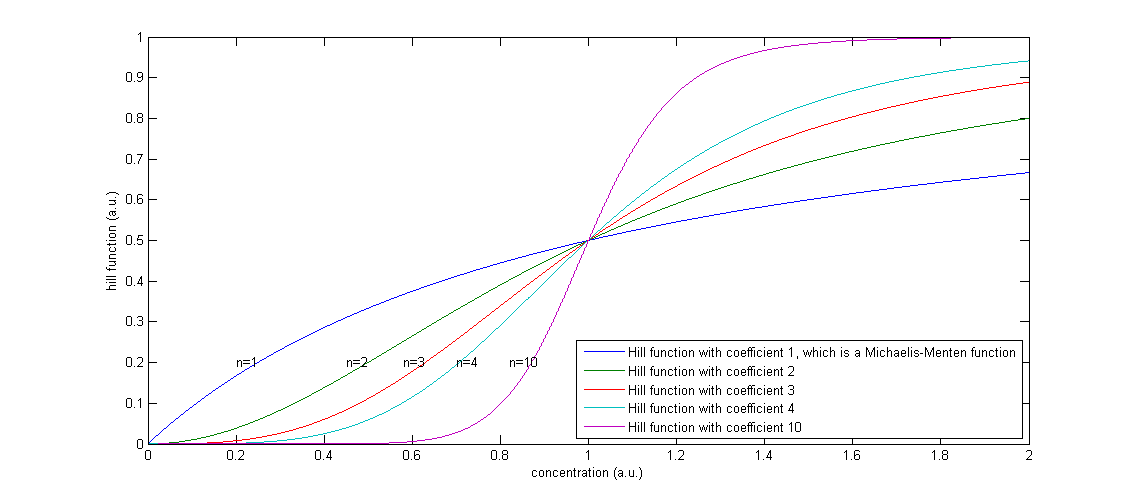Figure 2: The effect of a higher Hill coefficient

The other parameters in this model are the maximal production rates, which are indicated by β’s and the decay rates, which are indicated by α’s. The degradation term is proportional to the concentration of that component, in which the proportionality constant α can be seen as the percentage of the present proteins that degrades in one unit of time.
We will now discuss the different rate equations and how they are linked to the logical circuit of Figure 1. The concentration of each of the components changes because of a production and a degradation term. The production depends on the presence of the other components and the degradation is as described above. Specifically for A the production is activated in the presence of D and repressed in the presence of C. The logical AND gate can be seen as if both components have to give a positive signal (presence of D and absence of C) in order to have production of A. The format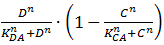exhibits this behaviour; when C is above its threshold the second term equals zero, when D is absent the first term gives zero. Consequently both D has to be present and C has to be absent to have a non-zero production term. X is a simpler case, since its production is only influenced by A; when there is a sufficient amount of A, there is production of X. C is subject to an OR gate and in order to model this we use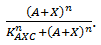. When either A or X (or a combination of the two) reaches the required level there is production of C. For the other three rate equations the same explanation holds since it is a symmetric system.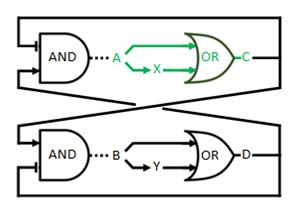Figure 1: Logical circuit displaying our oscillator

##### 1.2.2 Enzymatic production of quorum-sensing molecules

As mentioned on the design page the quorum-sensing molecules are produced by an enzymatic step. Equation 2 shows the equations that result in that behaviour. The transcription factors control the production of the enzymes rather than A and B directly. The production of A and B is modelled by using Michaelis-Menten kinetics (SA/(KA+SA)). To not overly complicate our model we will assume that the substrates SA and SB are at saturation levels. This means they are a lot higher than KA, KB respectively and the Michaelis-Menten consequently returns 1. This resulting system of equations is shown in Equation 3.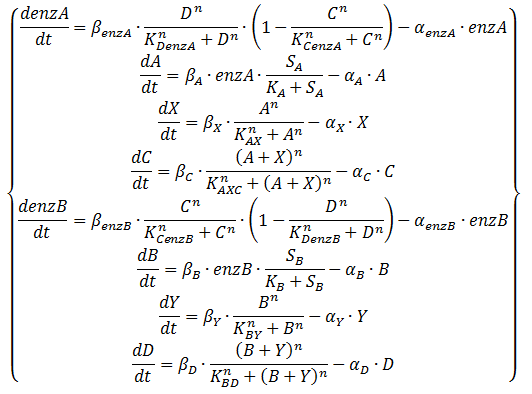Equation 2: ODE system exhibiting the behaviour of the oscillator, including an enzymatic production step for the QS molecules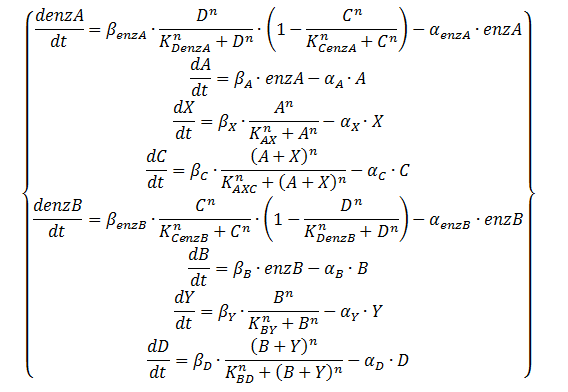Equation 3: ODE system exhibiting the behaviour of the oscillator, including an enzymatic production step for the QS molecules with the assumption of saturation of those enzymes

##### 1.2.3 Alternative OR gate

In the part before we used an actual OR gate, however, in the wetlab we use an approximation of a real OR gate. We implement the gene for production of C (D) twice, once with a promoter that is induced by A (B) and once with one that is induced by X (Y). This is easier to implement although the behaviour is somewhat different. There is now a higher expression when both the inducers are present. There is no difference in the fact that this displays a asymmetric time delay, of which the importance is discussed in the design page. This altered circuit is displayed as Figure 3.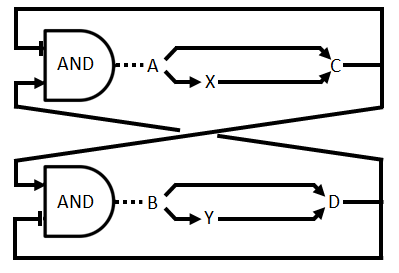Figure 3: Logical circuit displaying the our oscillator with enzymatic production of the QS molecules and the alternative OR gate

This is translated into Equation 4 by using the format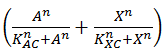instead of. It is clear that this will return 1 in the case that either A or X are present, 0 when both are absent and 2 when both are present.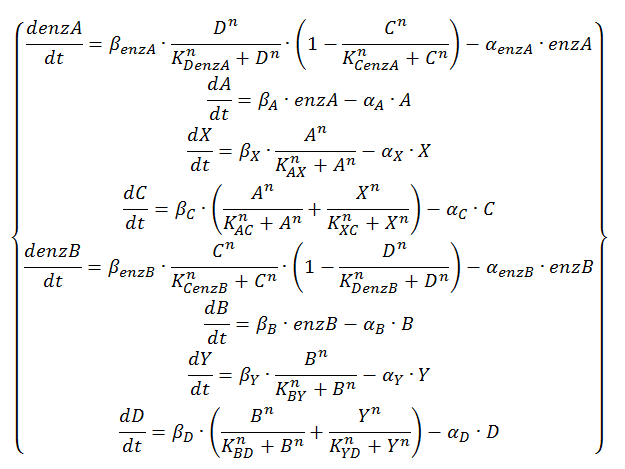Equation 4: ODE system exhibiting the behavior of the oscillator with enzymatic production of QS molecules at substrate saturation and the alternative OR gateFigure 4: The Flemish Super Computer Centre (click to visit their website)

#### 1.3 Matlab analysis

First we determined the ranges to which the parameters belong. This was done for the degradation rates (α), the production rates (β) and the thresholds (K). We chose random sets of parameters in these ranges and tested whether sustained oscillations take place. Because of the enormous parameter space we could not scan it entirely, but this method still proves whether the tested system inherently produces sustained oscillations. To conduct our computations we made use of the Flemish Super Computer Centre (VSC), which is a partnership of the five Flemish university associations. To use this we first had to rewrite the tests so they could be run in parallel on the supercomputer cluster at our university. Although this seemed straightforward, we encountered an obstacle in the generation of random numbers. When testing on the supercomputer, it appeared like we got 100 identical results in one test. The fault was rapidly determined: the way random number are generated in Matlab. By default, the seed Matlab uses to generate (pseudo)random numbers is always the same if this is the first command you execute. To solve this, we implemented a solution found on (walkingrandomly.com). This involved getting random numbers out of the Linux kernel random number generator and using these as a seed for the random number generator in Matlab. We tested several systems this way, starting with the simplest and building up to the final one. For each of these systems we checked how many of the tested sets of parameters produce oscillations. We used this to calculate the fraction of oscillating sets in the tested parameter space (see Box 1).

##### 1.3.1 Selection of the parameter range

For the degradation rates of the proteins we assume they follow the N-end rule and are not in one of the unstable categories. This means the dilution rate controls the effective degradation rate and thus we use values that are appropriate for that. More information on how these values have been found can be found on the methyl salicylate modelling page. The ranges can be found in Table 1.

A steady-state concentration of proteins can be easily determined by setting each of the rates to zero. In the case of maximal production rate, the rate equation has the following form: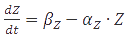and when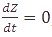, the equation can be easily converted to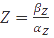, which gives the steady-state concentration of Z, or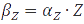, when all but the production rates are known.
In the case of metabolites, that are produced by enzymes the rate at full production capacity equals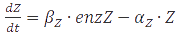which becomes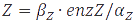at steady-state. When the enzyme is at its own steady-state concentration during maximal production rate this becomes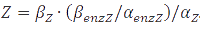.

The maximal production rates (β) of the proteins can vary enormously, for instance due to differences in copy number. However, the goal of this oscillator is to have the components in the chromosomal DNA of the cells. We thus assume values that are in the rage of production rates of chromosomal genes. For those ranges we use the assumption that the steady-state concentrations of proteins is equal to β/α (see Box 2), we used the α’s as determined above and we use protein abundances as determined by Ishihama et al. (2008). They determined a steady-state concentration for enzymes to be around 65 per cell and for components of the biosynthetic machinery to be around 105. An important remark is that the assumption that steady-state concentrations are equal to β/α is only correct for as long as the production is fully on and not when there were some inhibitory effects active. This means that the lowest protein abundances that are determined by Ishihama et al. can be a lot lower than β/α due to for instance regulation of the production rate, which was consequently not at its maximum. When the production rate is only half of its maximum for instance, the steady-state concentration of the protein is only equal to 0.5∙β/α instead of β/α, which means the lowest values determined by Ishihama et al. be too low. On the other hand the highest values might also be too high, since we are not dealing with components of the biosynthetic machinery. It thus seems wiser to us to discard the extremes and only probe between 6.5∙102 and 104. We will use these values and transform them to production rates by using the formula from Box 2 and the degradation rates from Table 1. The calculated values can be found in Table 1.

The maximum production rates due to the enzymes cannot be determined this way, but also span an enormous range. Shaefer et al. (1996) found proof, however, that enzymes that produce quorum-sensing molecules have a low maximum production rate, and they found specifically for the Vibrio fischeri LuxI protein that β equals 1.1 molecule per minute. Since our model is not confined to one enzyme, we will again test a bigger scope. We will again use a range of two decades for this parameter, as can be seen in Table 1.

The final parameters we will look into is the threshold value. Our method will consist of randomly picking production rates degradation rates of metabolites. As mentioned in Box 2 the steady-state concentration at full production capacity equals β/α for proteins and , which means there is no possibility of having a higher level of that protein or metabolite. For the proteins we will use this as an upper bound for setting the possible threshold, since the threshold otherwise would never be reached. We will assume the threshold is reached somewhere in the process, but this is not necessary to have production as long as the Hill coefficient is smaller than infinity. As a lower bound we will allow one decade of variance. The formulas used are displayed in Table 1. The steady-state concentration at full production capacity of metabolites can however vary significantly from the thresholds of the proteins that act as transcription factors. Since the threshold is in reality a function of the interaction between promoter and transcription factor and not of the steady-state concentration of the transcription factor, it would be unfair to use such different values for thresholds for proteins on the one hand and thresholds for metabolites on the other. Instead we will use an the median of all β/α of the proteins for the thresholds for metabolites. Since we use a uniform probability distribution for both α and β the median will be the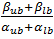. The resulting value is shown in Table 1, with a lower bound that is one decade lower.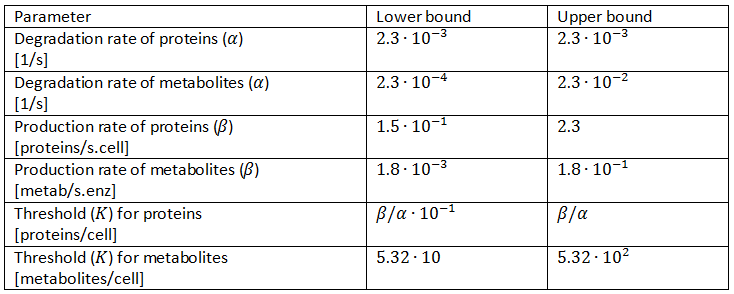Table 1: Parameter ranges
##### 1.3.2 Setup of the in silico experiments

We have already skimmed the fact that we will use random probing of the parameter space in order to establish the fact that sustained oscillations take place. Here we will discuss how we exactly probed the parameter space, what we took as initial conditions for the ODE solver and how we tested for oscillating behaviour. Since we cannot assume any probability distribution, we will stick to a uniform distribution for each of the parameters. Each of the parameters will be allowed to vary independently from one another, except for the protein degradation rates and the metabolite degradation rates. Those will all be chosen to be the same since the value is based on the growth rate of the colony, which is of course the same from the perspective of different proteins within that same colony.

The initial conditions determine quite a lot of the behaviour of the network when there are non-linear functions involved. In order to test whether the system behaves as predicted we will use initial conditions that correspond to the explanation on the design page. This means we start with a high concentration of A and zero of each of the others. It is now only necessary to determine what qualifies as a high enough concentration. For this we use the maximum of KAX and KAC as a point of reference because that can be seen as the threshold for A to have an impact. We simply chose twice that threshold, which should suffice to spark the system.

Detection of oscillating behaviour was done differently for tests involving ODEs and PDEs. The latter had to be checked manually, because of their often very different appearance. The ODE tests however were checked on stable oscillations by a self-written script. This program takes the Fourier transform of the signal after the transient part using the Matlab command for Fast Fourier Transform (FFT). The the components of this FFT are split into the zero frequency component and all other components. Then, the sum of the absolute values of the components with a nonzero frequency is compared to the absolute value of the zero frequency component. If this ratio is greater than one, the signal is marked as oscillating. Practically, this means that the signal has to have some periodic components that are of the same order of magnitude as the zero frequency component, which is the average of the signal. Signals with negative values were automatically discarded due to their physical impossibility. If any of the signals in a test run was oscillating, the whole test run was marked as positive.

As an output we will determine what the oscillating fraction of the tested parameter space is. This is a good measure to find out how easily the system reaches oscillations. Since we randomly probe throughout the parameter space and find a binary result of either oscillating or either not. As this is a Bernoulli trial process, we can calculate the 95% confidence interval of the fraction of the parameter space that oscillates. The formula displayed as Equation 5 gives that 95% confidence interval and according to Brown Cai and DasGupta (2001) this formula should function well as long as both n∙p > 5 and n∙(1-p) > 5. This means that only in the case of a fraction close to 0 or close to 1 there can be a problem. However, when it is that close to either of the extreme values, the results are already clear enough because it simply means the system hardly ever oscillates, or oscillates almost all the time. When p is as close to 1 that n∙p > 5, a maximum 4 out of 100 tests produced a non-oscillatory system and we can definitely say that the system easily produces oscillations in that parameter space.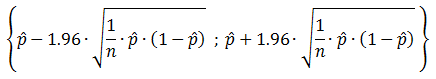Equation 5: 95% confidence interval for the proportion of success in a Bernoulli trial process.
##### 1.3.3 Testing the preliminary model

A first test using the preliminary model, which is shown in Equation 1, did not frequently give oscillations. This is easily explained because of the fact that the thresholds, who were chosen of the same order of magnitude as the theoretical maximum, were never approached. The reason the levels of the components do not reach that high level is because of the many direct and indirect inhibitory effects within the system. Those inhibitory interactions keep the production rates, and thus the concentrations, well below their theoretical maximum. Since the thresholds are far from reached, this also means there is no activation of subsequent components and thus the whole process of oscillating does not get sparked. As a control for this hypothesis we tested how the oscillating fraction of randomly picked parameter sets varies when different ranges for thresholds are used. We did this by taking only a fraction of β/α as the upper bound and again a lower bound that is one tenth of the upper bound. This has been done each time for a small pool of only 100 randomly chosen sets of parameters and the results are shown in Table 2. From an upper bound of 0.05∙β/α onwards oscillations are far from rare and further lowering of the thresholds does not change that feature.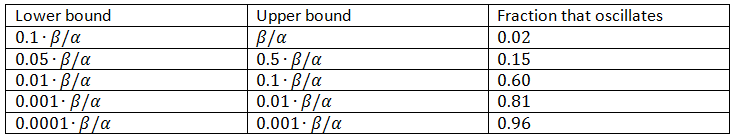Table 2: Effect of threshold height

We will thus use a slightly altered parameter space and continuously test several of them. We will let the thresholds vary from 0.01∙β/α to 0.1∙β/α, 0.001∙β/α to 0.01∙β/α and 0.0001∙β/α to 0.001∙β/α in three different tests. This also changes the thresholds for metabolites accordingly and these new parameter spaces are displayed in Table 3.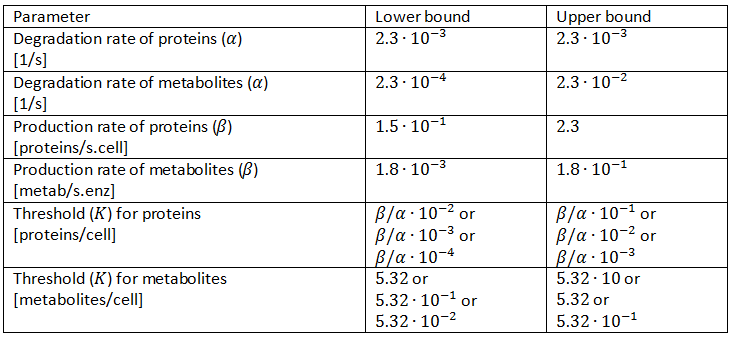Table 3: Revision of the parameter space

The different resulting behaviours for the situation in which the protein thresholds are allowed to vary from β/α∙10-3 to β/α∙10-2 are shown in Figure 5. The thresholds for metabolites do not matter thus far, since, there are no metabolites present in the preliminary system. Only A, X and C are shown since B, Y and D have a similar behaviour. There are of course differences since the production rates and thresholds are all varied independently of each other, but they add no extra qualitative information. In this figure we also left out the initial transient behaviour and only shows from 10,000 seconds till 50,000.

Figure 5 a to d shows the prevailing behaviour, which are oscillations in which C reaches a lot higher levels than A or X. Figure 5 a shows a typical example of such oscillations and in Figure 5 b this has been zoomed in on A and X. Figure 5 c and d show another interesting example of oscillations, in which C is of the same order of magnitude as the others. This only happens a few times, which is probably due to the fact that C has two means of activation, but none of inhibition. This makes the production of C more intense and last longer. The specific equations we used probably also have a big effect on this, as well as the chosen parameter ranges. Another remark that can be made is that the levels of A and B are also low in absolute terms. Strictly speaking, this is not bad, since we made a lot of assumptions which mean quantitative features like the amplitude and frequency are unreliable. As mentioned before we only want to assert the qualitative features of this model like oscillations and synchronization. We can now already see that in this simple case oscillations do occur and after the discussion of Figure 5 we will give a confidence interval of the fraction of the parameter space in which oscillations occur.

The occurrence of oscillations like the one displayed in Figure 5 c and d are not qualitatively different from those from Figure 5 a and b. Though they allow for a better description of how the several components relate to one another. It shows that the production of C goes on for an extended period even after the disappearance of A, which is the effect of the coherent type 1 feed forward loop. This feature can be easily related to the presence of X even after A has disappeared.

The other type of behaviour this model showed was reaching a steady state. An example of this is shown in Figure 5 e. In this image the system starts with oscillations in C that are damped, but it can also be that the steady-state is reached without ever oscillating. This is of course unwanted behaviour, but regardless of the parameter set used, there are always initial conditions that make the system reach a steady-state. In a heavily non-linear system of equations like ours, there are always some of those steady-states that are stable.

Theoretically there are two other types of behaviours possible in the case of continuous non-linear equations. The first possibility is extinction, which is a steady-state situation in which all of the components their levels become and remain zero. This behaviour has only been observed when using thresholds that are way too high. The second other possibility is an explosion of the system. Then one or more of the components’ level rises indefinitely. This type of behaviour is not possible in the systems we will study, since each component has a degradation term that is proportional to the level of that component and the production term is not and has a maximum value.

Figure 5: The resulting behaviour | a, the typical oscillations, b, the same as a but zoomed in at A and X, c, oscillations in which the difference between A and X on the one hand and C on the other are not so different, d, the same as c but zoomed in at 4 periods, e, an acquired steady-state, f, a steady-state in which each component returns zero.

We then sought the fraction of parameter sets that result in oscillations for each of the three situations. The resulting confidence intervals for that fraction can be found in Table 4. None of the confidence intervals overlap each other, so lower threshold ranges significantly increase the fraction of oscillations, which supports our explanation that oscillations could not occur due to the fact that the thresholds could not be reached. The oscillating fraction is high, showing that this system of equations inherently produces oscillations.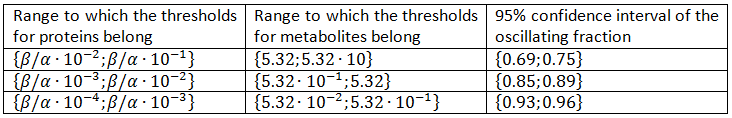This shows that this simple system creates sustained oscillations, which is not that remarkable on its own, since non-linear systems usually have some sets of parameters and initial conditions that make it produce oscillations. There is also no proof that once the threshold ranges are low enough to be reachable the oscillating fraction of the differs by altering those ranges. This is good news since those ranges are hard to predict and apparently they do not have a big influence on the occurrence of oscillations. In what follows we will check different systems and see how easily they reach oscillations.

##### 1.3.4 Testing the other ODE systems constructed earlier

We made confidence intervals for the fraction of the parameter space that has oscillations on the other representations of our model as well. We again used the parameter ranges from Table 3 and let each of the parameters vary independently from one another.

The first of the systems we test here is displayed as Equation 3. Here we add enzA and enzB which are responsible for the production of A and B (that are now metabolites instead of proteins). We do this in order to come closer to the proposed implementation of our model. Another thing that is important to mention here is the fact that this extra step means an extra delay in the system. This is useful because in an oscillating system the different peaks should not have too much overlap. This perhaps counters the fact that we did not resort to DDE’s. This should benefit the occurrence of oscillations. The entirely different ranges of production and degradation of the metabolites (here A and B) in respect to the proteins that act as transcription factors (here X, C, Y and D) may put a strain on the occurrence of oscillations, because this makes their ranges of concentrations also differ enormously. This means that problems may occur when we use the same order of magnitude as thresholds, because we determined earlier that they should at least be of reachable height. On the other hand, we do not allow pre-determined differences in parameters (e.g. using entirely different threshold ranges for proteins than for metabolites), since it has been our purpose all along to create a system that oscillates for a broad range of different in vivo components (e.g. promoters). It would thus be unfair to already determine that thresholds for metabolites have to be 100 times as high as for proteins for instance, because the mechanism they work with does not differ per se.

We did the same test as earlier in order to know the fraction of the parameter space that produces oscillations and the results are shown in Table 5. These results show a significant increase with respect to those in Table 4, with fractions that are close to 1, indicating that this system is an oscillator. This proves that the extra delay benefits the oscillating behaviour of the system.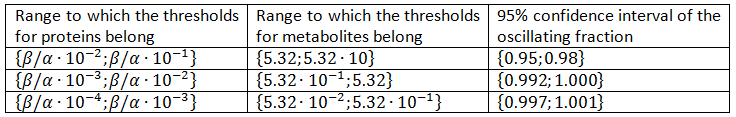Table 5: Confidence interval of the oscillating fraction of the parameter space of the system displayed as Equation 3.

In the next step we added an alternative version of the OR gate, of which the resulting system is shown as Equation 4. The resulting confidence intervals are shown in Table 6 and exhibit no significant differences with the results in Table 5.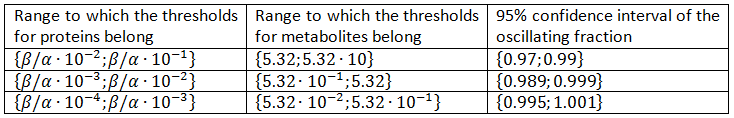Table 6: Confidence interval of the oscillating fraction of the parameter space displayed as Equation 4

Each of the models show a very frequent occurrence of oscillations, proving that our proposed system inherently produces oscillations. A recurrent phenomenon was the big difference in amplitude of the different components. However, we chose not to go in depth on this topic and which component’s oscillations had the highest amplitude was not always the same. A second noteworthy feature is the type of oscillations that occur, namely relaxation oscillations. This is a type of oscillations in which the production shifts when thresholds are reached. This is exactly what Hill functions represent and thus circadian rhythms, a widespread biological oscillator, often exhibit this type of oscillations (Maheshri and O’Shea, 2007).

#### 1.4 Conclusion

As required our system produces sustained oscillations for a very broad range of parameters. We simply used broad ranges for each of the parameters and the oscillations followed easily. There was only one parameter our used ranges were not backed up that strongly, namely the threshold value K. However, the system produced oscillations as long as the value was reachable, so its value is not crucial for the production of oscillations. Of course the production of oscillations in its own is not an impressive feature, since many known networks do that, as for instance the important example proposed by Elowitz and Leibler (2000).

The features of the proposed system that result in synchronization and rapid synchronization are undoubtedly the most important. An explanation of how this exactly happens can be found on the design page. Here we will show how we tested these properties. When we tested the parameter space for oscillations, we could stick to one cell only. Now, however, we will need spatial heterogeneity, which expresses the fact that there is ‘empty’ space in between cells.

In order to test whether synchronized oscillations occur, we will use a colony of ten different cells of which each has different parameters. This allows us to test whether synchronized oscillations occur even though there is cell-to-cell variability. This phenotypic variability can for instance arise due to differences in their position in the cell cycle and thus in overall protein production rates. A second feature we will test here is the occurrence of rapid resynchronization. This will be tested by using again a colony of ten different cells, however the difference will now lay in the level of each of the chemical species (A, X, C, B, Y and D) inside the cell. This expresses the differences that arise due to stochastic effects (Kærn et al., 2005). This is relevant because at any time a cell can express each of the proteins at an entirely different level than the others. We require that even then they rapidly resynchronize, which is an inherent feature of our model.

Each of the two tests will be conducted with a multitude of oscillating parameter sets which we received from the procedure above. As for the reaction rates we will stick to Equation 4 since this is the most complete system and it is this one we are eventually interested in. To test whether oscillations occur we use the same strategy as before.

#### 2.1 Constructing the PDE’s

The space we will study contains ten cells which are 16 µm apart from each other. This corresponds to a moderate cell density of 2*108 cells/ml (Park et al., 2003). We will take the cells to have a diameter of 1 µm and since we study 10 cells the total size of the space we study will be 160 µm in total. The variable that will display the location will be x and ranges from 0 to 160 µm. We will implement both a diffusion and a reaction term, of which the latter will contain a production and a degradation term. Only the diffusion term is entirely new here and is displayed as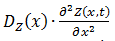. Equation 6 displays the entire system, in which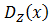displays the diffusion constant and reactionZ(x,…) the reaction term. The dependence on the position of the diffusion constant can be explained by the fact that all of the components except for A and B cannot diffuse out of the cells and consequently the diffusion constant is zero at the cells their borders for every component except for A and B. The dependence on the levels of the different components of the reaction term is explained above, the dependence on the position of the reaction term is due to the fact that there is only production inside of the cells. The only components that are present outside of the cells are the colony-wide molecules. We assume there degradation takes place there as well, in the assumption that they degrade through spontaneous degradation or dilution, which can also be a simplified representation of other types of degradation. For the reaction term it is also interesting to emphasize that we do use Equation 4 for this. However, the production term will be different inside and outside of the cells, whereas the degradation term will be uniform across the studied space and we thus actually divide Equation 4 in a production and a degradation term.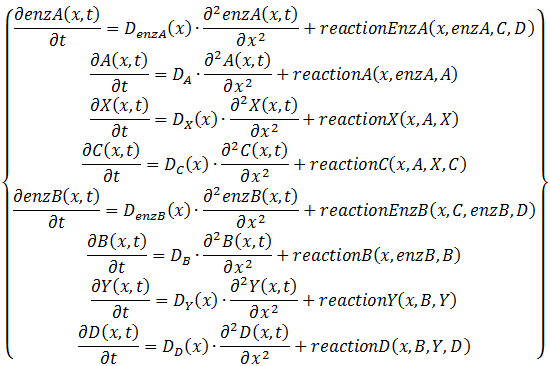Equation 6: System of PDE's displaying our system in a living colony

After solving this system for the first time we found discrepancies near the border of the cells (not shown). This had to do with the discontinuity in the functions reactionZ(x,…) and. We solved this problem by using splines, which serve as continuous alternatives. This is shown in Figure 6 a for the production rate and in Figure 6 b for the diffusion constant. We only used splines for the production part of the reaction term, since the degradation term is equal throughout our studied space. We will not elaborate further on the subject of splines since their only use to us is making a solution without irregularities possible. Figure 6 a also shows nicely how the total production rate does not change, since the surface underneath each of the two curves is equal.

Figure 6: Difference between splines and step functions | a, The relative size of production inside and outside of a cell using step functions or splines to model the transition. b, The relative size of the diffusion coefficient inside and outside of a cell using splines to model the transition.

We will use several sets of oscillating parameters to test both of the synchronization features of our model and for the diffusion coefficient of the metabolites we will use the one already mentioned on the design page, namely DN-(3-Oxododecanoyl)-L-homoserine lactone, which equals 4.9 ∙ 102 µm2/s (Stewart, 2003). The diffusion coefficient of the proteins will be chosen to be several orders of magnitude higher since they are a lot higher and are hindered a lot in there movement through the cell.

One last remark about the model used is about the threshold values. As determined earlier using β/α is unreachable and we thus resorted to 0.1∙β/α. Since we have some empty space in between the cells, this means the production of the colony-wide molecules is actually spread out over eight times the size of a cell. Consequently, we chose to divide all of the thresholds by eight as well, in order to have a similar situation as before. In fact the most similar situation would be reached if only the thresholds for A and B would be changed, but as mentioned before it is unfair to have predetermined differences in the ranges of parameters for one component than for another when there is no decent explanation.

#### 2.2 Synchronization in a phenotypically heterogeneous population

Since we are aiming for synchronized oscillations, even in a highly variable cell population, we have to take the cell-to-cell variability into account. This variability arises even in a genetically identical population due to noisy expression of genes. In order to test whether our model can cope with this, we let each of the ten cells have different parameters. We conducted this test for 100 randomly probed parameter sets of each of the threshold categories. We represented cell-to-cell variability by letting each of the maximum production rates (β) vary according to a normal distribution (without allowing negative values).

The results of this test can be found in Table 7, where the 95% confidence intervals for the fraction of populations that produce synchronized oscillations regardless of the cell-to-cell variability that is allowed. The fraction that produces synchronized oscillations is significantly lower in the case of a higher relative variance, an effect that vanishes when the threshold ranges are lower. Another meaningful comparison is with the results from Table 6. This comparison shows that the fraction of oscillations is mostly significantly lower in when cell-to-cell variability is allowed. However, even in the case in which most variability was allowed, the lowest fraction of synchronized oscillations was well over 50% and in almost each of the situations the upper boundary of the 95% confidence interval was close to 1. This shows that the system tested here produces synchronized oscillations despite of cell-to-cell variability.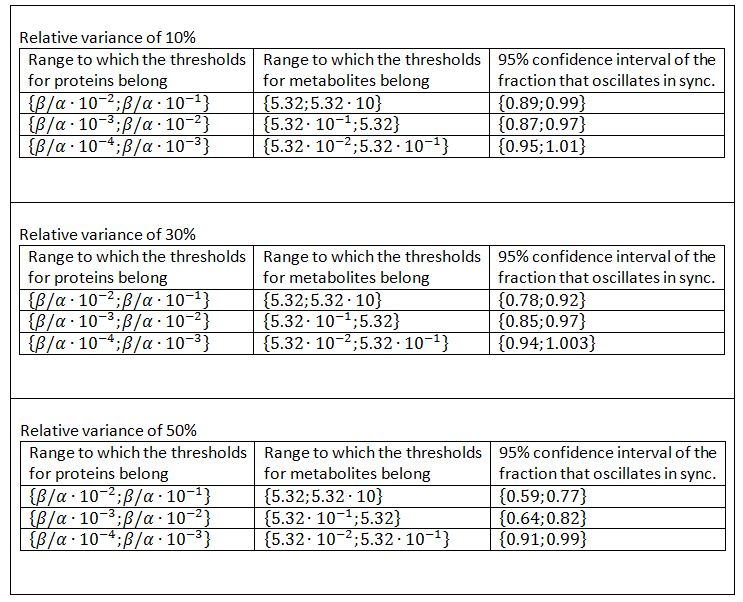Table 7: Confidence intervals of the fraction that produces synchronized oscillations when cell-to-cell variability is taken into account.

Figure 7: An example of synchronized oscillations when cell-to-cell variability is allowed|a, The oscillations of A in an example in which 10% relative variability is allowed, b, The oscillations of C in an example in which 10% relative variability is allowed, c, The oscillations of A in an example in which 50% relative variability is allowed, b, The oscillations of C in an example in which 50% relative variability is allowed.

Figure 7 shows an example of synchronized oscillations when differences in parameters for the different cells are allowed. Figure 7 a and b show how it looks when 10% relative variability is allowed. Figure 7 a shows A and Figure 7 b shows C. Clearly the oscillations of those components are synchronized throughout the studied space. Figure 7 c and d show this with 50% relative variability and Figure 7 d obviously gives a more extreme image, however the oscillations remain synchronized. Figure 7 c even shows that A still oscillates evenly throughout the studied space. The second one is obviously more extreme and consequently there are fewer oscillations in that case, but the data in Table 7 clearly show that synchronized oscillations continue to occur frequently.

#### 2.3 Rapid resynchronization

The last test we conducted is testing whether rapid resynchronization occurs. We do this by having completely different initial conditions for a number of the cells and test whether oscillations still occur. We will each time let X and C be four times as high in some cells and Y and D be one fourth compared to the other cells. We chose to let 40% of the cells have these different values since this is a very extreme test and we want to seek the borders of what’s possible. In Figure 8 and Figure 9 we show two examples in which a different group of cells started out of sync. These examples show that there is no real difference in speed of resynchronization and we will simply take the setup of Figure 9 in our tests. Important to note is that the resynchronization happens incredibly fast, which is very beneficial for an in vivo oscillator.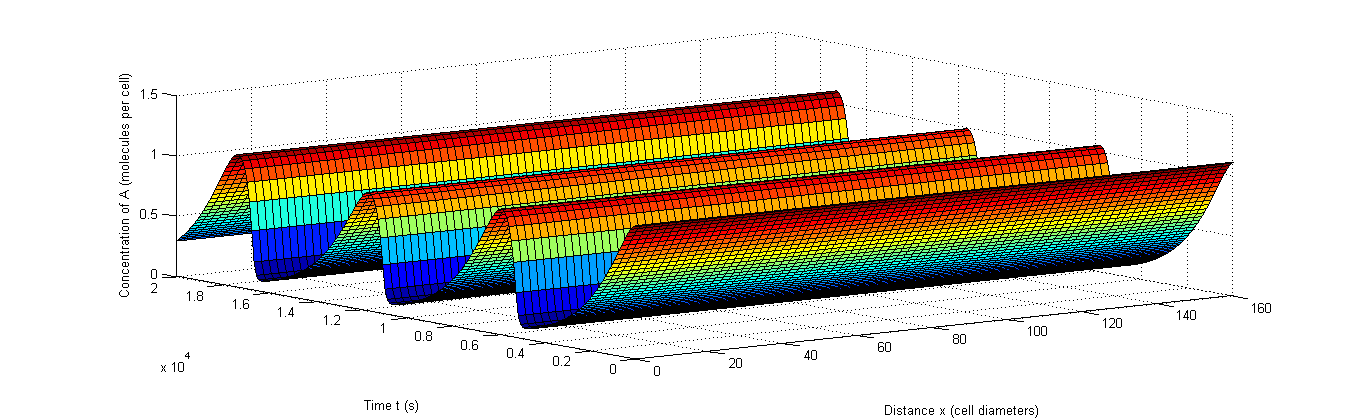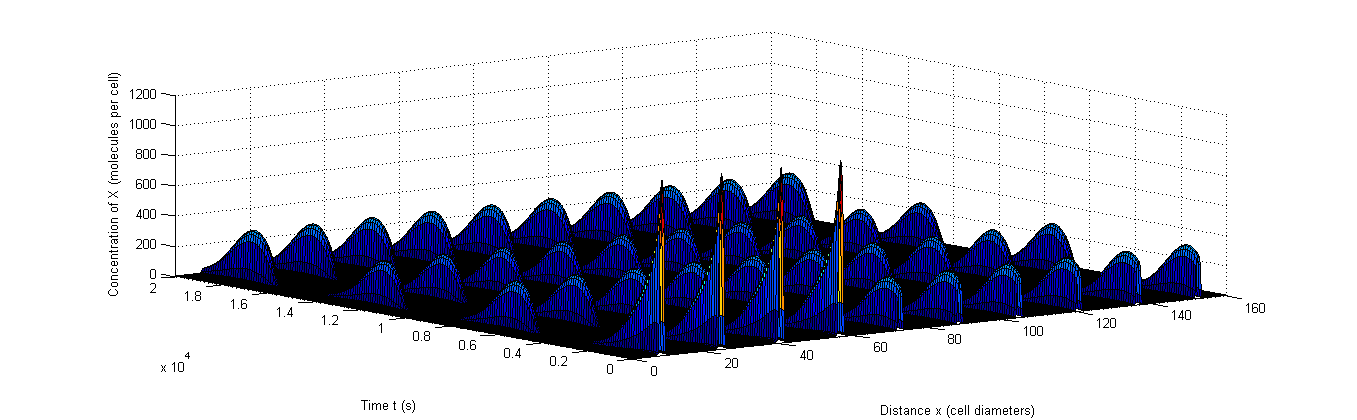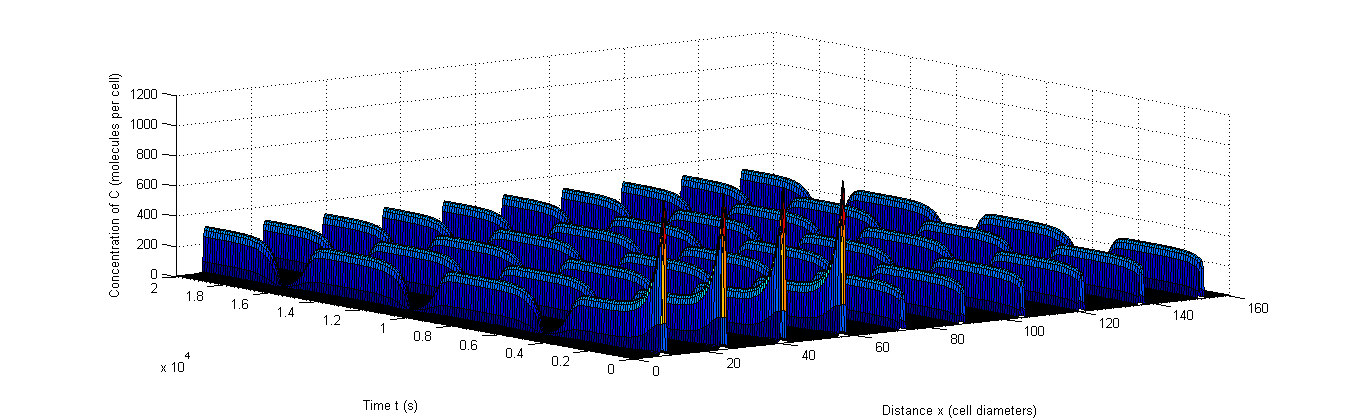Figure 8: Example of rapid resynchronization in which the first 40% of the cells start out of sync. |a, The fluctuations of A, b, The fluctuations of X, c, The fluctuations of C.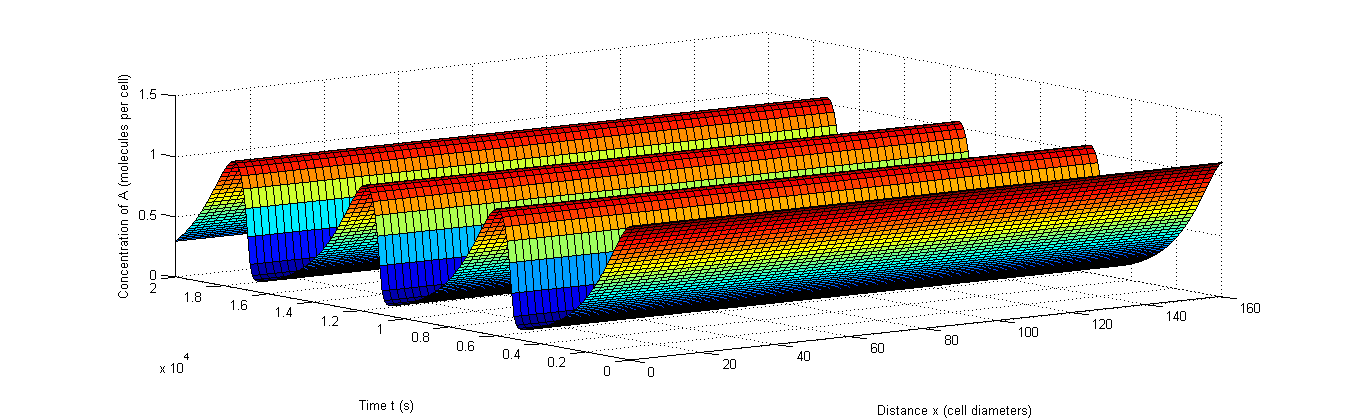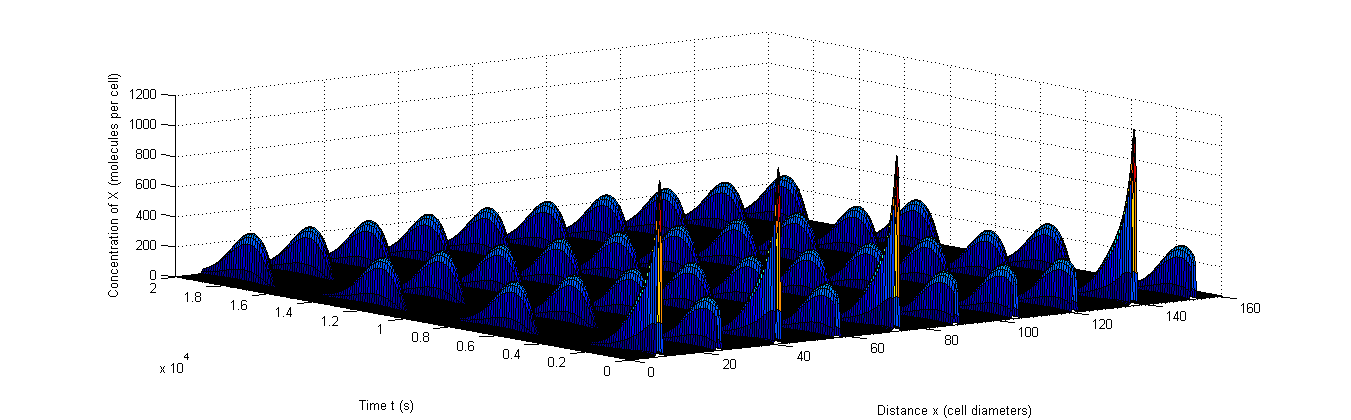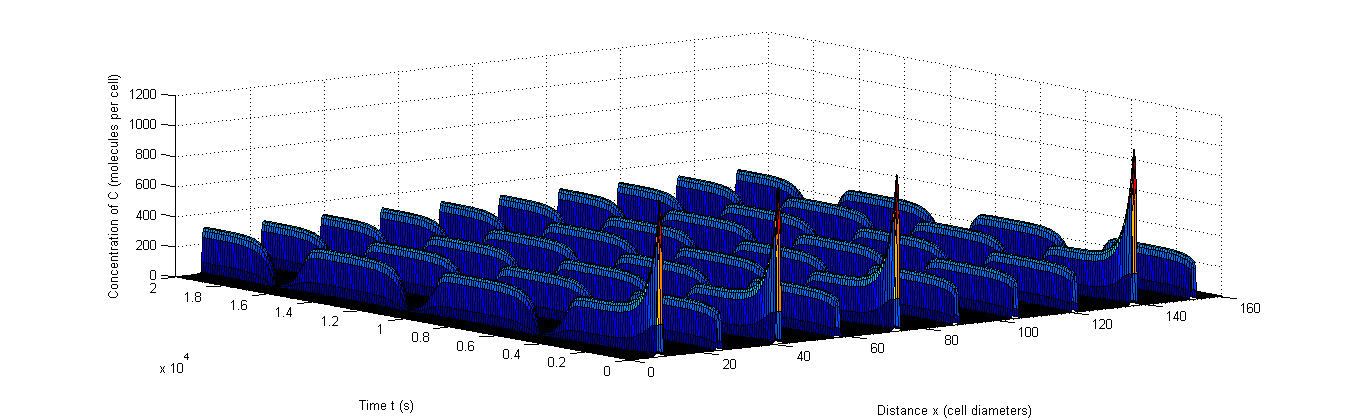Figure 9: Example of rapid resynchronization in which the 40% of cells that start out of sync. are put on positions 1, 3, 5 and 9.|a, The fluctuations of A, b, The fluctuations of X, c, The fluctuations of C.

We’ve tested this for 100 randomly probed parameter sets in each of the threshold ranges discussed earlier. The results of this test are shown in Table 8 and except for the second threshold range, the confidence intervals overlap with those of Table 6, which shows the oscillating fractions when only one cell is studied. This, together with the very high fractions of oscillations shows that the studied system exhibits rapid resynchronization, as is crucial for any colony wide oscillator.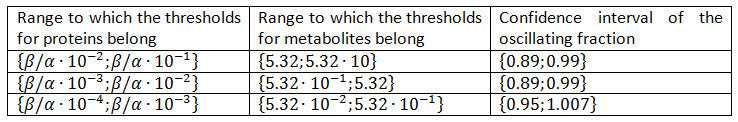Within the range of reasonable parameters, a high fraction resulted in oscillatory behaviour. This is required for three reasons. First of all this means that a broad range of components can be used to create our model in vivo, which was our goal all along. Secondly it is important to have oscillations occur in a number of different cell densities, since this influences the metabolism of the cells in many ways. Example given: the production of the colony-wide molecules is spread out over a bigger volume when cell densities are low than when they are high. Thirdly, this means that oscillations will still occur even if parameters change due to an extra load on the cell’s system, which is for instance the case if we couple a production module to our oscillator.

The oscillator clearly can withstand a high amount of cell-to-cell variability, which is an aspect that is obviously very important in synchronized oscillations. The rapid resynchronization is also a feature our model has and this is important in the context of the stochastic effects desynchronise the cells. Our results also showed that the resynchronization occurs incredibly fast, which is very beneficial in order to have sustained oscillations.

The several tests conducted on our model gave satisfactory results and indicate our system creates synchronized oscillations. However, extrapolating this to saying it will also work in vivo is a bridge too far. This is because there is an enormous amount of complexity we did not take into account. We did use the most widespread technique for studying genetic networks, namely through ODE’s (Polynikis, Hogan and di Bernardo, 2009) so our results show it is definitely worth testing it in vivo. For this test the first steps have been taken in our wetlab page about the subject.

Brown, L. D., Cai, T. T., DasGupta, A. (2001). Interval Estimation for a Binomial Proportion. Statistical Science, 16(2):101-133.
Danino, T. et al. (2010). A synchronized quorum of genetic clocks. Nature, 463:326-330.
Ishihama, Y. et al. (2008). Protein abundance profiling of the Escherichia coli cytosol. BMC Genomics, 9:102.
Kærn, M., Elston, T. C., Blake, W. J., and Collins, J. J. (2005). Stochasticity in gene expression: from theories to phenotypes. Nature Reviews Genetics, 6:451-464.
Elowitz, M. B., and Leibler, S. (2000). A synthetic oscillatory network of transcriptional regulators. Nature, 403(6767):335-338.
Maheshri, N. and O’Shea, E. K. (2007). Living with noisy genes: how cells function reliably with inherent variability in gene expression. Annu. Rev. Biophys. Biomol. Struct., 36:413-434.
Park, S. et al. (2003). Motion to form a quorum. Science, 301:188.
Polynikis, A., Hogan, S. J., and di Bernardo, M. (2009). Comparing different ODE modeling approaches for gene regulatory networks. Journal of Theoretical Biology, 261:511-530.
Rosenfeld, N. et al. (2005). Gene Regulation at the Single-Cell Level. Science, 307(5717):1962-1965.
Schaefer, A. L., Val, D. L., Hanzelka, B. L., Cronan, J. E. JR., and Greenberg E. P. (1996). Generation of cell-to-cell signals in quorum sensing: Acyl homoserine lactone synthase activity of a purified Vibrio Fischeri LuxI protein. PNAS, 93:9505-9509.
Stewart, P. S. (2003). Diffusion in biofilms. Journal of Bacteriology, 185:1485-1491.
Tyson, J. J. in Computational Cell Biology (eds. Fall, C. P., Marland, E. S., Wagner, J. M. and Tyson, J. J., 2002), 230-260 (Springer, New York).